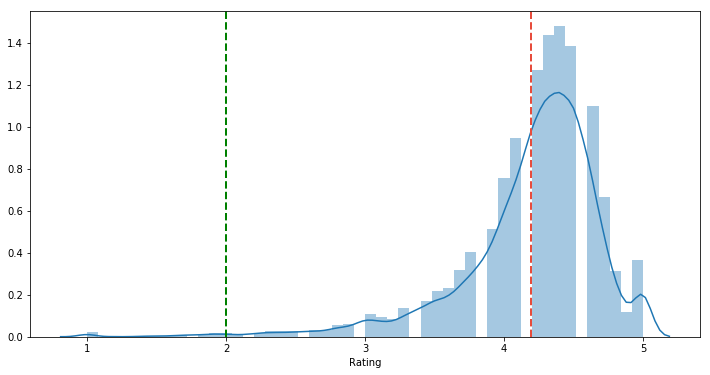# Estimates of Location

Last updated: January 13th, 2020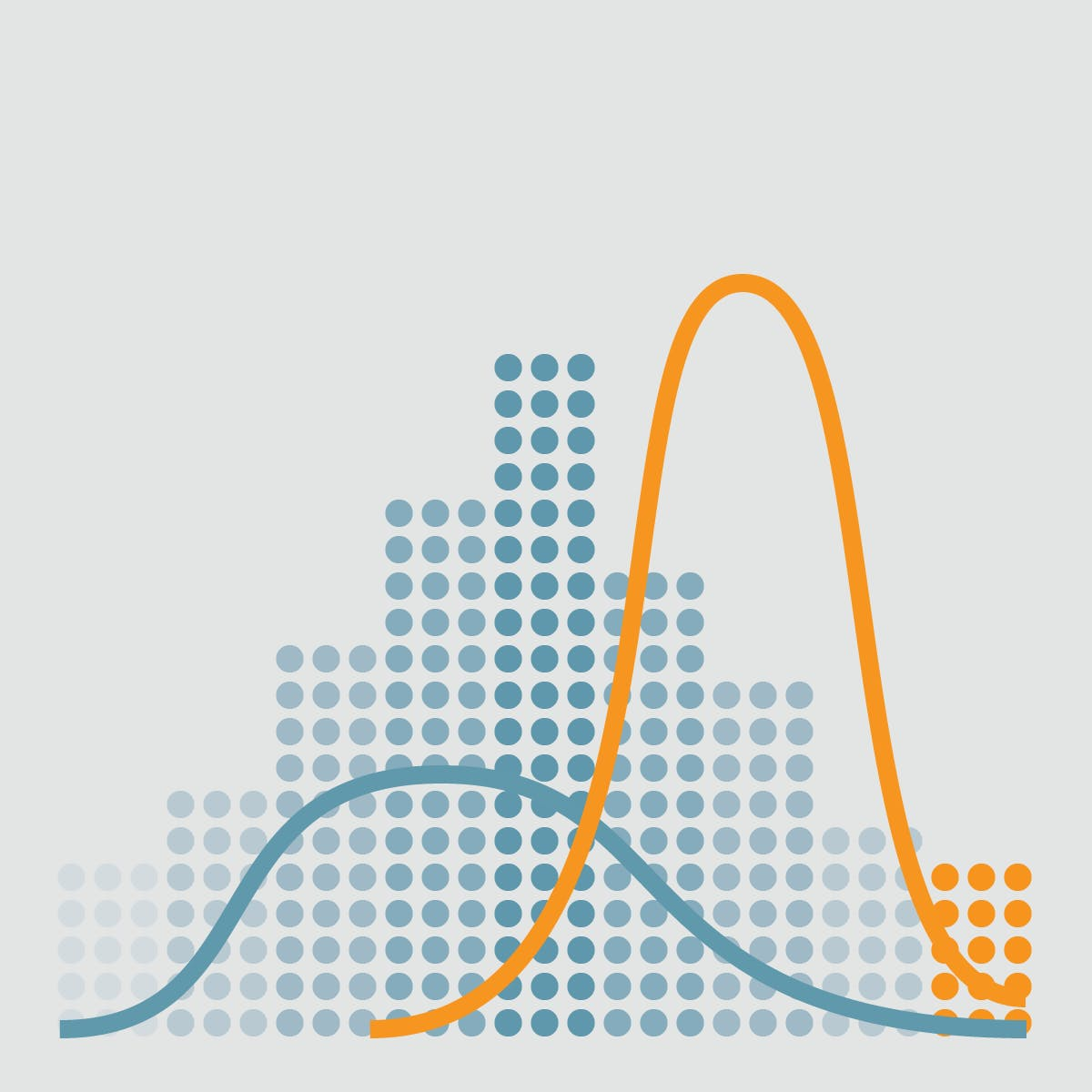# Estimates of Location¶

Variables with measured or count data might have thousands of distinct values. A basic step in exploring your data is getting a "typical value" for each feature (variable): an estimate of where most of the data is located (i.e., its central tendency).

👉 External resource: Jake Vanderplas' keynote about Statistics for Hackers at PyCon 2016: https://www.youtube.com/watch?v=Iq9DzN6mvYA## Hands on!¶

In :
import numpy as np
import pandas as pd
import matplotlib.pyplot as plt
import seaborn as sns

%matplotlib inline


We'll use the following Google Play Store Apps dataset in this lesson:

In :
df = pd.read_csv('data/googleplaystore.csv')


Out:
App Category Rating Reviews Installs Price Content Rating Genres Android Ver
0 Photo Editor & Candy Camera & Grid & ScrapBook ART_AND_DESIGN 4.1 159 10,000+ 0 Everyone Art & Design 4.0.3 and up
1 Coloring book moana ART_AND_DESIGN 3.9 967 500,000+ 0 Everyone Art & Design;Pretend Play 4.0.3 and up
2 U Launcher Lite – FREE Live Cool Themes, Hide ... ART_AND_DESIGN 4.7 87510 5,000,000+ 0 Everyone Art & Design 4.0.3 and up
3 Sketch - Draw & Paint ART_AND_DESIGN 4.5 215644 50,000,000+ 0 Teen Art & Design 4.2 and up
4 Pixel Draw - Number Art Coloring Book ART_AND_DESIGN 4.3 967 100,000+ 0 Everyone Art & Design;Creativity 4.4 and up## Measuring Central Tendency¶

Mathematically, central tendency means measuring the center or distribution of location of values of a data set. It gives an idea of the average value of the data in the data set and also an indication of how widely the values are spread in the data set.

That in turn helps in evaluating the chances of a new input fitting into the existing data set and hence probability of success.

#### Frequency distribution of a variable¶

The first thing we're going to do is plot a line of every sample value of the Rating column:

In :
df.info()

<class 'pandas.core.frame.DataFrame'>
RangeIndex: 10840 entries, 0 to 10839
Data columns (total 9 columns):
App               10840 non-null object
Category          10840 non-null object
Rating            9366 non-null float64
Reviews           10840 non-null int64
Installs          10840 non-null object
Price             10840 non-null object
Content Rating    10840 non-null object
Genres            10840 non-null object
Android Ver       10838 non-null object
dtypes: float64(1), int64(1), object(7)
memory usage: 762.3+ KB

In :
df['Rating'].head(10)

Out:
0    4.1
1    3.9
2    4.7
3    4.5
4    4.3
5    4.4
6    3.8
7    4.1
8    4.4
9    4.7
Name: Rating, dtype: float64
In :
df['Rating'].plot(color='#3498db', figsize=(12,6))

Out:
<matplotlib.axes._subplots.AxesSubplot at 0x7f3865945c18>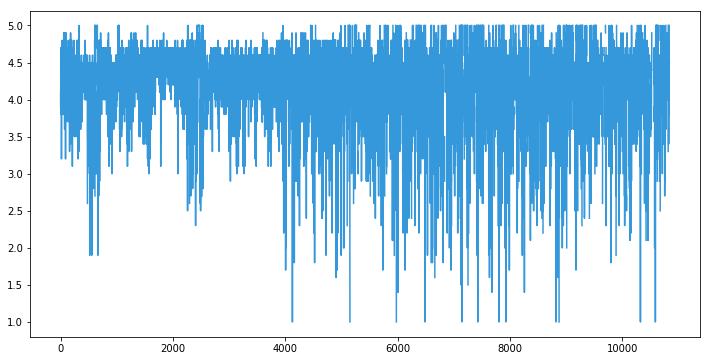### Histogram¶

It's a mess, so we're going to make an accurate representation of the distribution of the sample values by getting the frequency of each value.

In :
df['Rating'].value_counts().head(10)

Out:
4.4    1109
4.3    1076
4.5    1038
4.2     952
4.6     823
4.1     708
4.0     568
4.7     499
3.9     386
3.8     303
Name: Rating, dtype: int64
In :
freq = df['Rating'].value_counts().sort_index()

freq_frame = freq.to_frame()

freq_frame.plot.bar(color='#3498db', figsize=(12,6))

Out:
<matplotlib.axes._subplots.AxesSubplot at 0x7f38638a4978>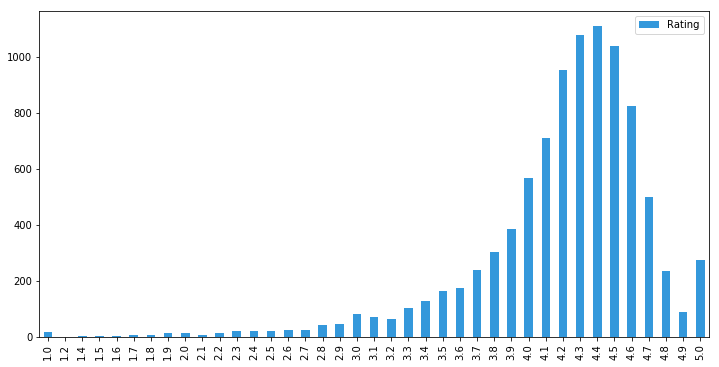This plot of the frequency (count) of the values is known as a Histogram:

In :
df['Rating'].plot.hist(bins=20, color='#3498db', figsize=(12,6))

Out:
<matplotlib.axes._subplots.AxesSubplot at 0x7f38637fd208>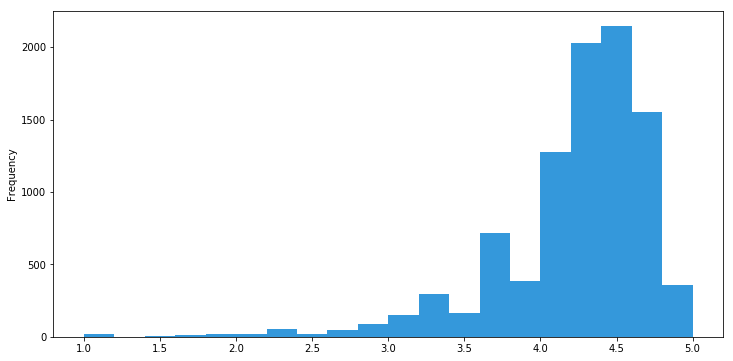In :
df['Rating'].plot.hist(bins=10, color='#3498db', figsize=(12,6))

Out:
<matplotlib.axes._subplots.AxesSubplot at 0x7f386370ff28>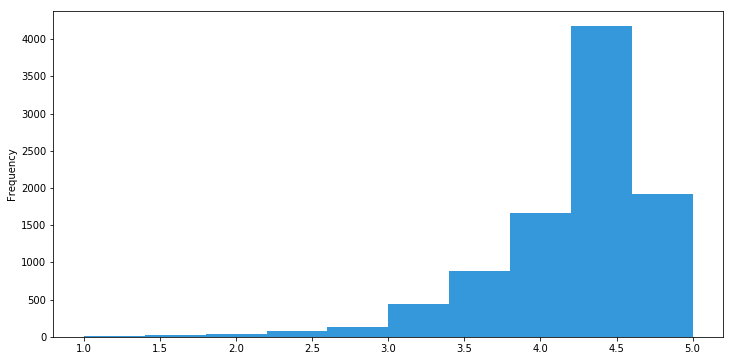## Density estimates¶

Related to the histogram is a Density plot, which shows the distribution of data values as a continuous line.

This density plot can be thought of as a smoothed version of a histogram, although it is typically computed directly from the data through a kernel density estimate.

We'll use Seaborn library to make our plots:

In :
plt.figure(figsize=(12,6))

sns.distplot(df['Rating'].dropna())

Out:
<matplotlib.axes._subplots.AxesSubplot at 0x7f38637d99b0>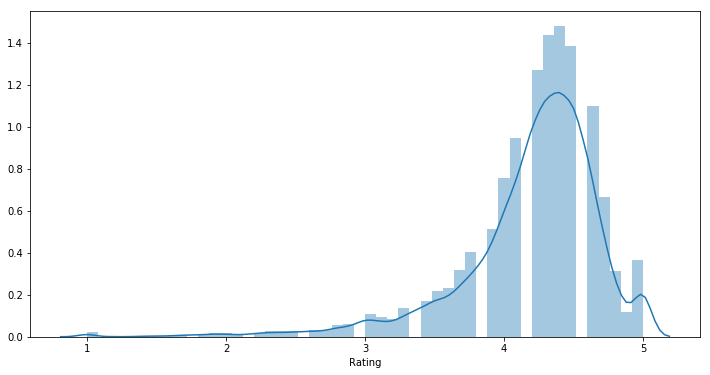## Mean¶

The sum of all values divided by the number of values. Also known as average. This is the most basic estimate of location.

The formula to compute the mean for a set of $n$ values $x_1, x_2, ..., x_n$ is:

$$Mean = \mu = \overline{x} = \frac{\sum\limits_{i}^n x_i }{n}$$

Let's calculate the mean of the Rating of Google Play Store Apps.

In :
list(df['Rating'])[0:15]

Out:
[4.1, 3.9, 4.7, 4.5, 4.3, 4.4, 3.8, 4.1, 4.4, 4.7, 4.4, 4.4, 4.2, 4.6, 4.4]
In :
mean_rating = df['Rating'].mean()

mean_rating

Out:
4.191757420456972
In :
plt.figure(figsize=(12,6))

sns.distplot(df['Rating'].dropna())

# Mean line
plt.axvline(mean_rating, color='#e74c3c', linestyle='dashed', linewidth=2)

Out:
<matplotlib.lines.Line2D at 0x7f38635e0a90>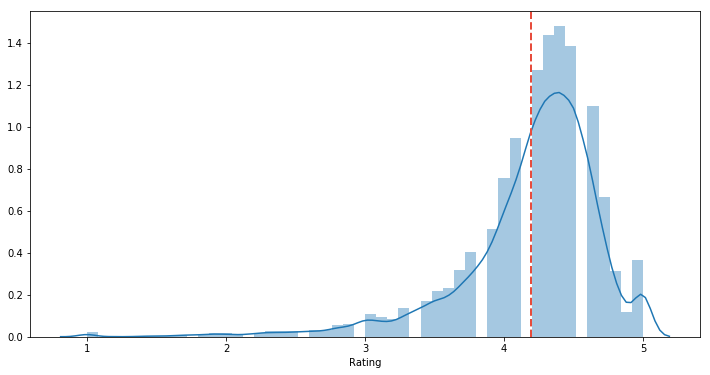#### Pros¶

• It works well for lists that are simply combined (added) together.
• Easy to calculate: just add and divide.
• It’s intuitive — it’s the number "in the middle", pulled up by large values and brought down by smaller ones.

#### Cons¶

• The average can be skewed by outliers — it doesn't deal well with wildly varying samples. The average of 100, 200 and -300 is 0, which is misleading.## Median¶

The value such that one-half of the data lies above and below. If there is an even number of data values, the middle value is one that is not actually in the data set, but rather the average of the two values that divide the sorted data into upper and lower halves.

$$6, 2, 9, 3, 13, 4, 9, 7, 12, 8, 10, 5, 13, 9, 3$$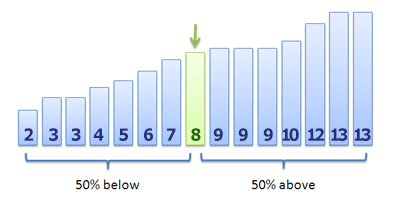Compared to the mean, which uses all observations, the median depends only on the values in the center of the sorted data.

The median will be a robust estimator of location since it is not influenced by outliers that could sked the results.

In :
numbers = [6,2,9,3,13,4,9,7,12,8,10,5,13,9,3]

np.median(numbers)

Out:
8.0

Now lets calculate the median for the Rating column of Google Play Store dataset:

In :
median_rating = df['Rating'].median()

median_rating

Out:
4.3
In :
plt.figure(figsize=(12,6))

sns.distplot(df['Rating'].dropna())

# Mean line
plt.axvline(mean_rating, color='#e74c3c', linestyle='dashed', linewidth=2)

# Median line
plt.axvline(median_rating, color='yellow', linestyle='dashed', linewidth=2)

Out:
<matplotlib.lines.Line2D at 0x7f38634b2978>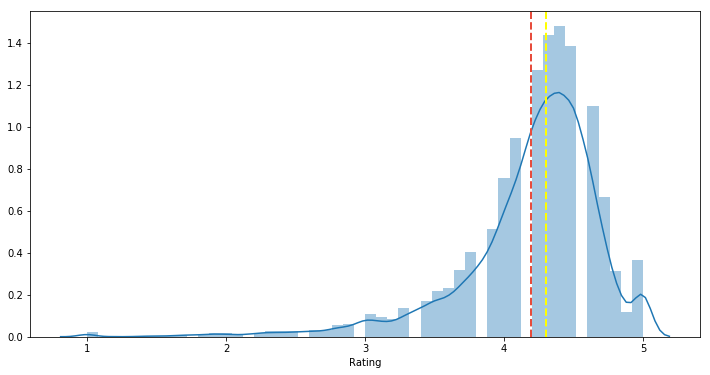## Mode¶

The most commonly occurring category or value in a data set.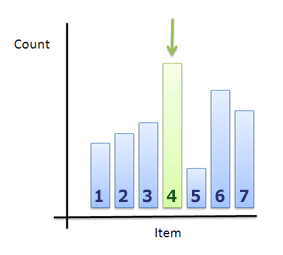In :
df['Rating'].value_counts().head()

Out:
4.4    1109
4.3    1076
4.5    1038
4.2     952
4.6     823
Name: Rating, dtype: int64
In :
mode_rating = df['Rating'].mode()

mode_rating

Out:
4.4
In :
plt.figure(figsize=(12,6))

sns.distplot(df['Rating'].dropna())

# Mean line
plt.axvline(mean_rating, color='#e74c3c', linestyle='dashed', linewidth=2)

# Median line
plt.axvline(median_rating, color='yellow', linestyle='dashed', linewidth=2)

# Mode line
plt.axvline(mode_rating, color='green', linestyle='dashed', linewidth=2)

Out:
<matplotlib.lines.Line2D at 0x7f3863332748>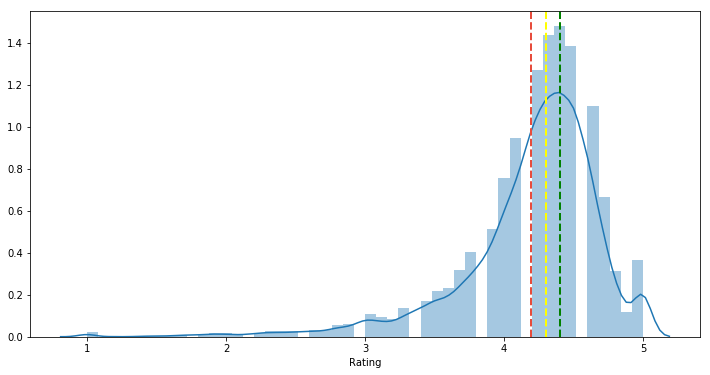👉 External resource: Khan Academy has a video showing an example of how to calculate Mean, Median and Mode: https://youtu.be/k3aKKasOmIw## Range and Mid Range¶

### Range (max - min)¶

In :
dist_range = df['Rating'].max() - df['Rating'].min()
dist_range

Out:
4.0
In :
plt.figure(figsize=(12,6))

sns.distplot(df['Rating'].dropna())

# Mean line
plt.axvline(mean_rating, color='#e74c3c', linestyle='dashed', linewidth=2)

# Range line
plt.axvline(dist_range, color='green', linestyle='dashed', linewidth=2)

Out:
<matplotlib.lines.Line2D at 0x7f38633a9ef0>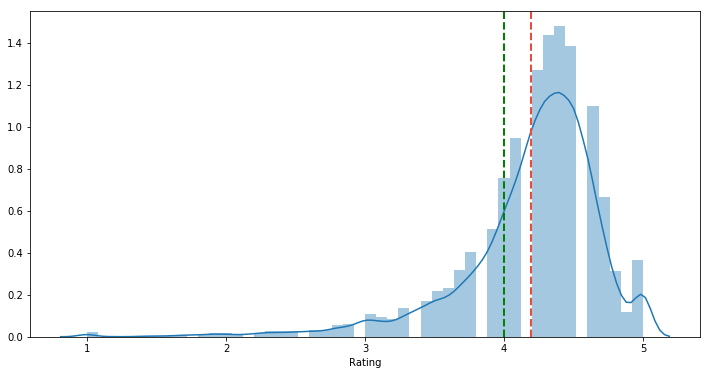### Mid range (range / 2)¶

In :
plt.figure(figsize=(12,6))

sns.distplot(df['Rating'].dropna())

# Mean line
plt.axvline(mean_rating, color='#e74c3c', linestyle='dashed', linewidth=2)

# Range line
plt.axvline(dist_range / 2.0, color='green', linestyle='dashed', linewidth=2)

Out:
<matplotlib.lines.Line2D at 0x7f3862d7b400>